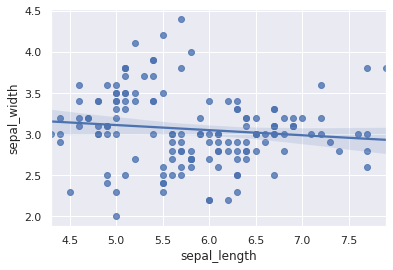## Basic Scatterplot with Seaborn

A scatterplot can be made using `regplot()` function of seaborn library. An example dataset from seaborn repository, iris dataset, is used in the example. The plot shows the relationship between sepal lenght and width of plants. In order to show the most basic utilization of this function, the following parameters should be provided:

• `x` : positions of points on the X axis
• `y` : positions of points on the Y axis

By default, a linear regression fit is plotted. It can be removed from graph by setting `fit_reg=False`.

``````# library & dataset
import seaborn as sns
import matplotlib.pyplot as plt

# use the function regplot to make a scatterplot
sns.regplot(x=df["sepal_length"], y=df["sepal_width"])

# make a scatterplot without regression fit
#ax = sns.regplot(x=df["sepal_length"], y=df["sepal_width"], fit_reg=False)

plt.show()``````## Contact & Edit

👋 This document is a work by Yan Holtz. You can contribute on github, send me a feedback on twitter or subscribe to the newsletter to know when new examples are published! 🔥